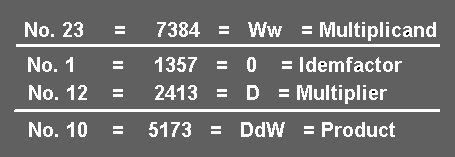The letters above the Exact Face numbers correspond to the turns -or tropes- from the zero (0) or Idemfactor face (1357, Upright Y Vex) performed to arrive at the viewed face. The 0 or Idemfactor is the beginning from which all the turns are based. To quickly refresh, these turns are: Major & Minor Widdershins (W&w) and Major & Minor Deosil (D&d).For example, Exact Face No.23 (7384, Prone O Vex) is reached by turning first a W followed by a w from the starting No.1 Exact Face (Idemfactor). Further, if we then make a D trope from No.23, we come to No.10 (5173, Supine Y Vex). Accordingly, we observe that the same trope (D) which turns No.23 into No.10 can also turn No.1 into No.12.

This is expressed as a Proportional Ratio or Equality by stating that No.1 is to No.12 as No.23 is to No.10. Or put in terms of an equation, No.23 times (x) No.12 equals (=) No.10 x No.1 (Idemfactor). This ratio would be diagrammed as shown below.Now, given the procedure for determining the cubic proportions through the Idemfactor (Exact Face No. 1), we can always calculate the the result (Product) without having to actually make the turns if the two faces are expressed properly as Multiplicand and Multiplier. This mathematical procedure is known as the Tropic Algorithm. The Tropic Algorithm consists of filling in the four Exact Faces as shown in the example and then establishing their proportional relationship through the consecutive tropes in order to arrive at the result.

NEXT